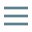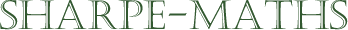# Glossary of terms

## E

•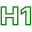### Equation

•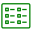An equation is a mathematical sentence. It is a way of showing that one expression is equal to another expression.

An equation should be thought of as a balance. The two sides of the equation must always be equal, so when we are manipulating equations, it is essential that we perform the same (allowable) operations to both sides of the equals sign.

•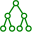Here are 2 equations. Note that there is always an expression either side of an equals sign.

$2x+3=11$

$x^2 - x -6=0$

•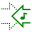•### Exponent

•An exponent is another name for a power or index. In the expression $x^3$, the $3$ is the exponent, index, power or order of the expression.

Exponents are a shorthand for repeated multiplication, in much the same way as multiplication is a shorthand for repeated addition.

•$p \times p \times p \times p \times p = p^5$

In the expression, $r^{-3}$, the exponent of $r$ is $-3$.

••### Expression

•An expression is the mathematical equivalent of a phrase or clause in standard language. It is not usually a complete sentence unless it is part of an equation or inequality. The simplest expression would be a term, which can be a number ($3$) or letter representing a number ($x$), or a combination of the two like  $2x$.

More complex expressions, like $2x^3 - 3x^2 + 4x -5$ can be made up from several terms connected with operators ($+ - \times \div$).

•Q: If an apple costs  $t$  pence, how much do 20 apples cost?

A: $20t$  pence.

Q: Simplify  $x +2x +4x$

A: $7x$

•